Related Articles

# Compound Inequalities

Compound Inequalities are the combination of two or more inequalities. These inequalities are combined using two conditions that are AND, and OR. These conditions have specific meanings and they are solved differently. The inequities in compound inequalities are individually solved using normal rules but the combinations of their answers depend on the AND and OR conditions. So, let’s start learning about the concept of compound inequalities including their solutions and various other solved examples as well.

## What is Compound Inequality?

A compound inequality is an inequality that combines two simple inequalities by either the “AND” condition or the “OR” condition.

The compound inequality with “AND” tells us that the condition is true when all the conditions in the compound inequality are true. Sometimes we do not even require to write AND and but it is understood. As in 2<x<4 it is nowhere written AND condition but the meaning of the above condition is x>2 and x<4. AND is also called the intersection of the solution sets for the individual statements.

Whereas “OR” indicates that, as long as either of the one statement is true, the entire compound sentence is true. It is called the union of the solution sets for the individual statements.

We also called AND condition a Conjunction and OR condition a Disjunction. Let’s compare both of these conditions using the following table.

## Compound Inequality Graph

Compound Inequality can be easily graphed in a coordinate plane and their solution is found using that. We should follow these points before graphing a compound inequality.

• The number on the right-hand side of the inequality is marked on the number line as in x < 3. 3 is marked on the number line.
• If the inequality is of the form <, and > we use an open bracket ( ) as it represents the number is not included. For example, in x < 3 we use the open bracket at 3 because it represents that 3 is not included in the inequality.
• If the inequality is of the form ≤, and ≥ we use a closed bracket [ ] as it represents the number is included. For example, in x ≤ 3 we use the closed bracket at 3 because it represents that 3 is included in the inequality.
• If the”>” or “≥” inequality is used we draw an arrow to the right side of the number in the number line.
• If the “<” or “≤” inequality is used, we draw an arrow to the left side of the number in the number line.
• In the final solution, we take intersection when the AND condition is given and union when the OR condition is given.

## Example of Graphing Compound Inequality with OR

The graph of inequality with the “OR” condition is represented by the union condition i.e. if either one statement is true, the entire compound sentence is considered to be true. The condition “OR” when utilized in a compound inequality forms what’s referred to as a Disjunction. We take the union of both the solution in OR compound inequality.

Example: Plot the interval x < 3 OR x > 5 on the number line.

Solution:

The solution to the above question is given in the image below,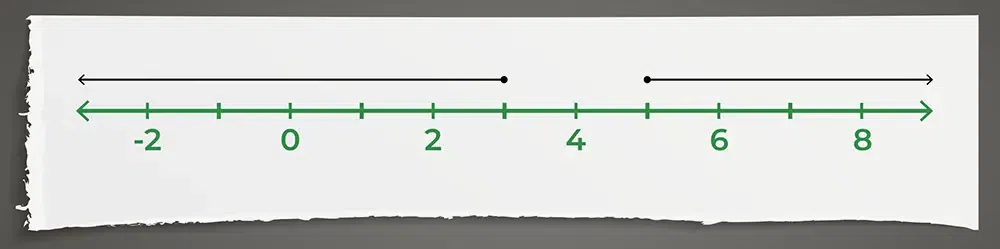The numbers 3 and 5 are not the solution to this inequality as the equality condition is not present in the above compound inequality.

## Example of Graphing Compound Inequality with AND

The graph of a compound inequality with the “AND” condition is represented by the intersection of the graph of the inequalities. The AND condition is true only when both conditions in the compound inequality are true. A compound inequality that uses the  “AND” is called a Conjunction. We take the intersection of both the solution in AND compound inequality.

Example: Grapg the condition on the number line x > 0 AND x < 4.

Solution:

The solution to the above question is given in the image below,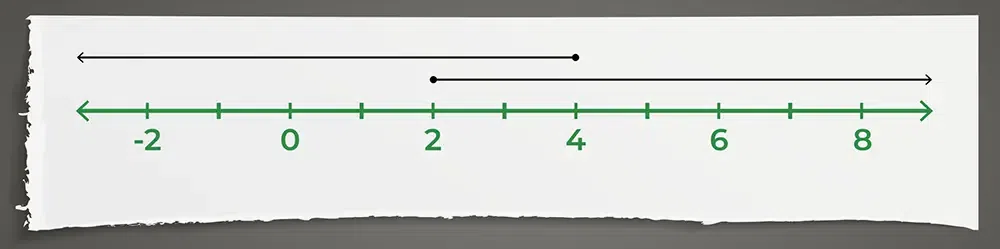So, in this case, 2 is a solution to the compound inequality, but 5 is not the solution because it only satisfies one of the inequalities, not both.

Note: We can also write the solution to the above compound inequality as,

0 < x < 4

## Solving Compound Inequalities

As it is already known that compound inequalities are formed when we merge two or more inequalities while solving compound inequalities firstly we solve the individual inequalities and then the union or intersection of the answers to those inequalities gives the solution of the compound inequalities which depend on the condition used, i.e. AND or OR conditions.

For solving the compound inequality follow the steps discussed below,

Step 1: Write the two inequalities individually from the compound inequalities.

Step 2: Solve each of the following inequality individually using the normal methods of solving inequalities.

Step 3: Mark the solution of both inequalities on the number line.

Step 4: If the compound condition is AND take the intersection of the solution and if the compounding is OR take the union of both the solution to get the desired solution of the inequalities.

### Example of Solving Compound Inequality with AND

Example: Solve the compound inequality 2 < 2x + 1 < 8

Solution:

Step 1: Mark the individual inequality from 2 < 2x + 1 < 8

2x + 1 > 2, 2x + 1 < 8

Step 2: Simplify both the inequality individually.

2x + 1 > 2

⇒ 2x > 2-1

⇒ 2x > 1

⇒ x > 1/2

AND, 2x + 1 < 8

⇒ 2x < 8 – 1

⇒ 2x < 7

⇒ x < 7/2

Step 3: Mark both solutions in the number line.

x ∈ (1/2, ∞)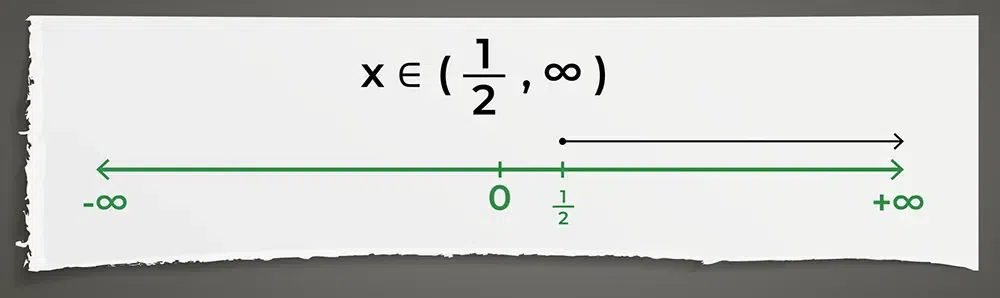x ∈ (-∞, 7/2)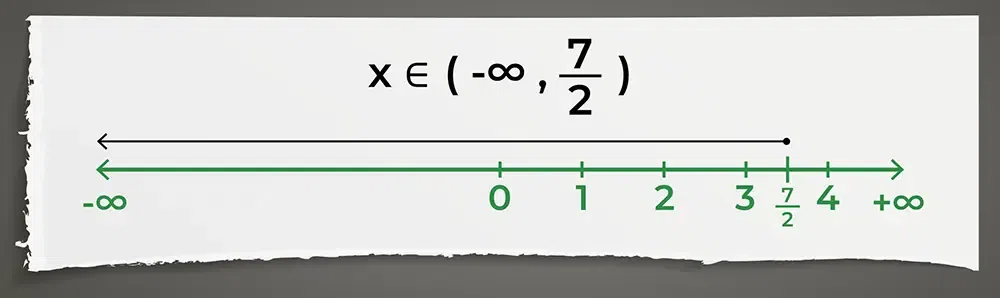Step 4: The compound condition is AND so we find the intersection of both the solution

x ∈ (1/2, ∞) ∩ (-∞, 7/2)

⇒ x ∈ (1/2, 7/2)

### Example of Solving Compound Inequality with OR

Example: Solve the compound inequality 2x + 1 > 8 OR x + 3 < 23

Solution:

Step 1: Mark the individual inequality from 2x + 1 > 8 OR x + 3 < 23

2x + 1 > 8, x + 3 < 23

Step 2: Simplify both the inequality individually.

2x + 1 > 8

⇒ 2x > 8-1

⇒ 2x > 7

⇒ x > 7/2

OR, x + 3 < 23

⇒ x < 23 – 3

⇒ x < 20

Step 3: Mark both solutions in the number line.

x ∈ (7/2, ∞)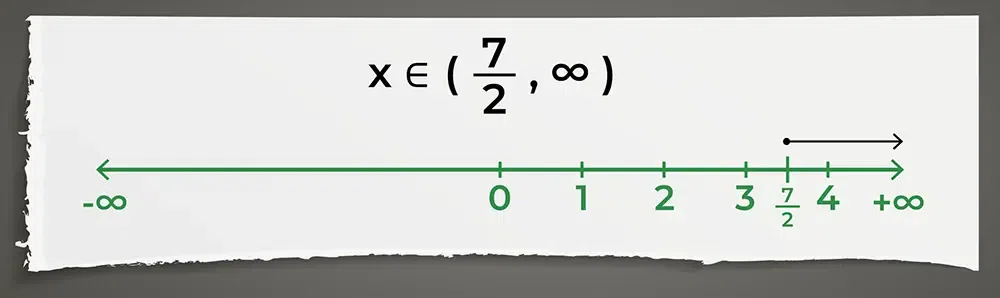x ∈ (-∞, 20)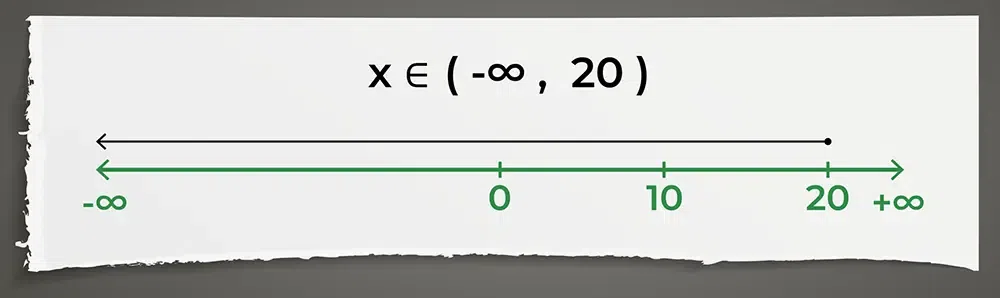Step 4: The compound condition is OR so we find the intersection of both the solution

x ∈ (7/2, ∞) U (-∞, 20)

## Compound Inequality Examples

Example 1: Solve for x in 2x+3 ≥ 7  OR  2x+9 > 11

Solution:

Given: 2x+3≥7  OR  2x+9>11

First Inequality 2x + 3≥ 7

⇒ 2x ≥ 7 – 4

⇒ 2x ≥ 4

⇒ x ≥ 2

⇒ x ∈ [2, ∞)

Second Inequality 2x + 9>11

⇒ 2x + 9 > 11

⇒ 2x > 11 – 9

⇒ 2x > 2

⇒ x > 1

⇒ x ∈ (1, ∞)

As the condition given is OR the solution to the compound inequality 2x+3 ≥ 7  OR  2x+9 > 11 is the union of individual inequality

The required solution is x ∈ [2, ∞) U (1, ∞) i.e. x ∈ (1, ∞)

Graphically this can be represented as,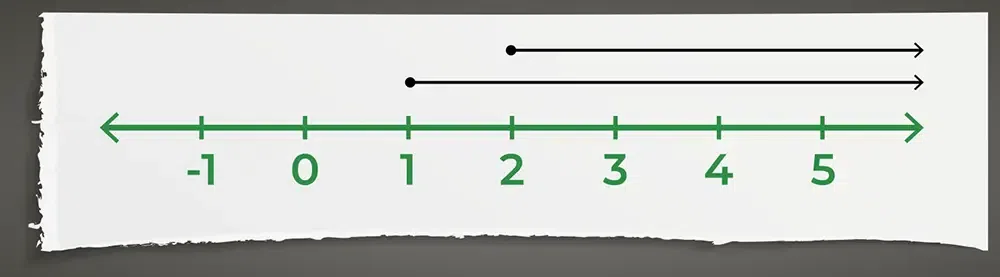Example 2: Solve for y in 2y + 7 < 13 OR −3y – 2 < 10

Solution:

Given: 2y + 7 < 13 OR −3y – 2 <10

First Inequality 2y + 7 < 13

⇒ 2y < 13 – 7

⇒ 2y < 4

⇒ y < 2

⇒ y ∈ (-∞, 2)

Second Inequality −3y – 2 <10

⇒ −3y <10 + 2

⇒ -3y < 12

⇒ -y < 4

⇒ y > -4

⇒ y ∈ (-4, ∞)

As the condition given is OR the solution to the compound inequality 2y + 7 < 13 OR −3y – 2 <10 is the union of individual inequality

The required solution is y ∈ (-∞, 2) U (-4, ∞)

Graphically this can be represented as,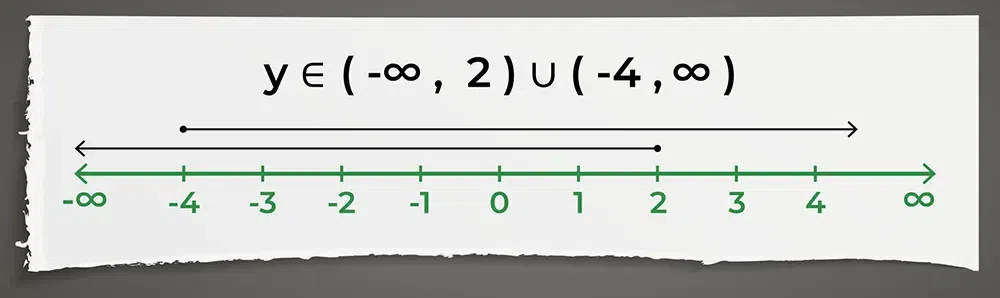Example 3: Solve for x: 3 x + 2 < 14 AND 2 x – 5 > -11.

Solution:

Given: 3 x + 2 < 14 AND 2 x – 5 > -11

First Inequality 3 x + 2 < 14

⇒ 3 x < 14 – 2

⇒ 3x < 12

⇒ x < 4

⇒ x ∈ (-∞, 4)

Second Inequality 2 x – 5 > -11

⇒ 2 x > -11 + 5

⇒ 2x > -6

⇒ x > -3

⇒ x ∈ (-3, ∞)

As the condition given is AND the solution to the compound inequality 3 x + 2 < 14 AND 2 x – 5 > -11 is the intersection of individual inequality

The required solution is x ∈ (-∞, 4) ∩ (-3, ∞)

Graphically this can be represented as,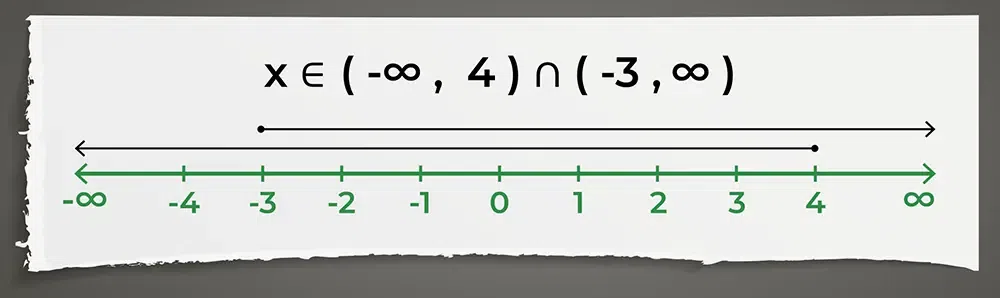## FAQs on Compound Inequality

### Q1: What are Compound Inequalities?

If two inequalities are combined using the “AND” or “OR” condition then it is called the compound inequality. We can understand it by the following example x > 3 AND x < 9. In this, we can say that all the values that are greater than 3 and less than 9 are answers to the above inequalities.

### Q2: What are examples of Compound Inequality?

Some examples of compound inequalities are,

• -17 < -4x – 2 ≤ 15
• 2x > 13 AND x < 9
• x>-3 OR 2x-4<5

### Q3: How to Solve Compound Inequalities?

Compound inequalities are solved separately and then the following changes are done,

• If the inequality is compounded by the AND condition we take the Intersection of both answers.
• If the inequality is compounded by the OR condition we take the Union of both answers.

### Q4: What is Compound Inequality Graph?

The compound inequalities graph is the graph that are drawn using the inequalities and the common region of all the inequalities is the required region of the compound inequalities.

### Q5: What does AND/OR mean in compound inequality?

In compound inequality AND/OR is used to define the intersection and union of the inequality.

• If we have to take the intersection of two or more inequality we use AND condition
• If we have to take the union of two or more inequality we use OR condition